Animated Physics - Atomic Orbitals of Elements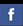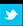Electron model
Proton/Neutron model
3D Atomic simulations

 Atomic Orbitals for S, P and D series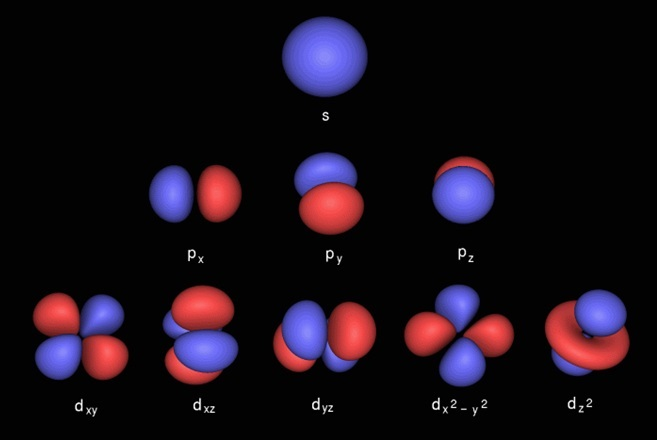Atomic orbitals shown are calculated from mathematical wave functions that predict the probability of finding an electron or a pair of electons in any specific region around the atom's nucleus. The shaded regions show where an electron is most likely to be. Up to two electrons can be found in each S-orbital shell shape. The P orbitals are orientated in a coordinate system with x, y and z directions where up to two electrons can be found in each direction for a total of six electrons in each P-level.

 Periodic Table
 The most un-reactive elements where electrons occupy all of the available energy slots at a particular level are found on the right hand edge. Helium has the first S-shell filled with two electrons. Neon has the first and second S-shell energy level filled together with the first P-shell for 10 electrons. Argon has the first, second and third S-shell energy level filled together with the first and second P-shell with 18 electrons.

 Atomic Orbitals in 2D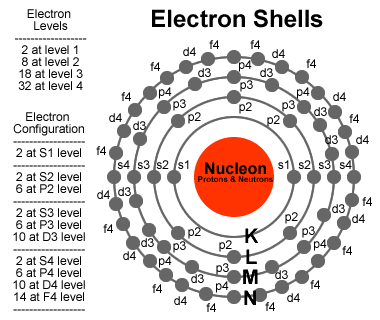Electrons in an atom possess four quantum numbers that shows what energy level the electron is in. Orbital, n=1,2,3... Azimuthal, l=0,1,2...n−1 Magnetic, ml=−l,−l+1...0...l−1,l and Spin, ms=−1/2,1/2 Pauli's exclusion principle says no two electrons can be in the same state. Starting with shell n=0, we have l=0 and ml=0, with ms=-1/2 or +1/2 (2 electrons). For shell n=1, starting with l=0, we have ml=0 and ms=-1/2 and +1/2 (2 electrons). Also for n=1, we have l=1 which allows ml=-1, 0 and 1 each state with ms=-1/2 and +1/2 (6 electrons).

Atomic Orbitals - Where are the electrons?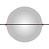S-level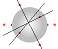P-level
Atomic orbitals are fundamental to the atomic orbital model (also called
the wave mechanics model or electron clouds), and provide a framework for visualization of the behavior of electrons. This model does not predict the exact location of the electrons but provides areas that electrons are most likely to be located in what is called an "electron cloud". Multi-electron atoms are built up from this model and provide an overall view and predictor of the properties of the atom. Repeating periodicity of blocks of 2, 6, 10, and 14 within the periodic table are due to the total number of electrons in a completed set of s, p, d and f atomic orbitals.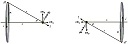The nucleon is represented as a circular shell. The first two electrons will align themselves on opposite sides of the proton, repelling each other. Since the electrons do not feel the proton pull inside the shell, they end on the edge of the shell. Over a short period of time, they align their spin with the proton, forcing the magnetic poles of the two electrons to face each other. These two electrons have opposite spins, face each other, and are the two members of the 1s energy level.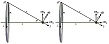An electron can be thought of as a closed current loop that has trapped an orthogonal magnetic field. Two circular current loops, located one above the other, with parallel planes, will attract each other, independent of the trapped magnetic field. Electrons pair up and combine their trapped magnetic fields, resulting in "bonded" electrons and lowered energy. In an element, this causes electrons to "stack up", where level-2s electrons will find a spot directly "above" the level-1s electrons relative to the center of the nucleon. For a teaching example of the attraction of two current loops using Ampere's law, Click Here.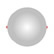Helium1s2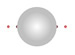Beryllium[He] 2s2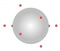Neon[He] 2s2 2p6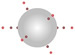Argon[Ne] 3s2 3p6
The six level-2p electrons, are at a slightly lower energy compared to the level-2s electrons and form a criss-cross pattern across the center of the nucleon. This pattern when viewed directly above one of the level-2p electrons, shows one electron directly below and the other four level-2p electrons forming a precise x/y grid. Next, the two level-3s electrons stack on the level-2s electrons and the six level-3p electrons stack on the level-2p grid. Although not discussed here, the next ten electrons are level-3d electrons and fill the 10 "holes" in the 18 electron Argon grid.

Simple bonding - Ortho and Para Hydrogen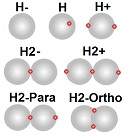Bonding between elements occurs when an electron, trapped in an energy level of one element, gets trapped in an energy level of another element, thereby binding the two elements together.

A hydrogen proton and an electron and is neutral in charge. Although neutral, the proton acting as a shell of charge with the electron close to the edge of the shell, will attract other neutral hydrogen atoms until one electron (or perhaps 2 in the ortho form) is trapped in both hydrogen shells binding them together. An electron trapping two protons together is called a para-bond and where two electrons trap two protons together, it's called an ortho-bond.

Click here to try playing a game where you aim and shoot an electron at other electrons bound to hydrogen protons. You see the effect of electrons interacting with other electrons and protons over attosecond (10^-18) timeframes and sub-nanometer (10^-9) distance scales.

Valence Electrons, bonding and Hybrid Orbitals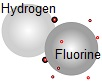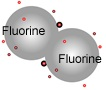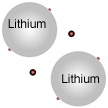Electrons in the outer shell are called "valence" electrons and are available for bonding. A shell of electrons that is one short, is said to have a "hole" in that spot. This attracts nearby electrons that may not yet be magnetically paired. This is modelled as a red dot (electron) filling a larger black circle (the hole). Bonds are formed by the overlapping of holes and electrons.

Hybrid orbitals form and stableize if the heavy nucleons temporarily form particular patterns. Electrons fill in the holes of an unstable pattern and over time stable hybrid orbitals form. like the sp orbital form when, for example in Fluorine, the 2pz orbital is available for bonding (the hole shown as a black spot) as is the 1s orbital of hydrogen (also in black). An electron in the level-2p hole of the Fluorine atom from the hydrogen atom, and an electron in the level-1s hole of the Hydrogen atom from the Fluorine atom, form a covalent bond between Hydrogen and Fluorine. Using a model called "Valence Bond Theory" to describe bonding molecules. Any spots missing electrons, can be considered "holes" that will attract an electron. This attraction forms the basis of bonding. sp, sp2, and sp3 hybrid orbitals In this model, bonds are formed by the overlapping of two atomic orbitals on different atoms. Hybrid orbitals, like the sp orbital form when, for example in Fluorine, the 2pz orbital is available for bonding (the hole shown as a black spot) as is the 1s orbital of hydrogen (also in black). An electron in the level-2p hole of the Fluorine atom from the hydrogen atom, and an electron in the level-1s hole of the Hydrogen atom from the Fluorine atom, form a covalent bond between Hydrogen and Fluorine.